Download Presentation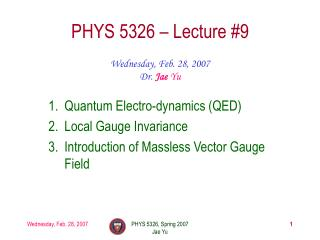PHYS 5326 – Lecture #9

# PHYS 5326 – Lecture #9 - PowerPoint PPT Presentation

PHYS 5326 – Lecture #9. Wednesday, Feb. 28, 2007 Dr. Jae Yu. Quantum Electro-dynamics (QED) Local Gauge Invariance Introduction of Massless Vector Gauge Field. Announcements. First term exam will be on Wednesday, Mar. 7 It will cover up to what we finish todayI am the owner, or an agent authorized to act on behalf of the owner, of the copyrighted work described.
Download Presentation## PHYS 5326 – Lecture #9

An Image/Link below is provided (as is) to download presentation

Download Policy: Content on the Website is provided to you AS IS for your information and personal use and may not be sold / licensed / shared on other websites without getting consent from its author.While downloading, if for some reason you are not able to download a presentation, the publisher may have deleted the file from their server.

- - - - - - - - - - - - - - - - - - - - - - - - - - E N D - - - - - - - - - - - - - - - - - - - - - - - - - -
Presentation Transcript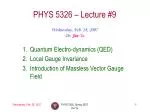### PHYS 5326 – Lecture #9

Wednesday, Feb. 28, 2007

Dr. JaeYu

• Quantum Electro-dynamics (QED)
• Local Gauge Invariance
• Introduction of Massless Vector Gauge Field

PHYS 5326, Spring 2007

Jae YuAnnouncements
• First term exam will be on Wednesday, Mar. 7
• It will cover up to what we finish today
• The due for all homework up to last week’s is Monday, Mar. 19

PHYS 5326, Spring 2007

Jae Yu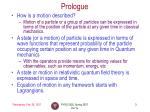Prologue
• How is a motion described?
• Motion of a particle or a group of particles can be expressed in terms of the position of the particle at any given time in classical mechanics.
• A state (or a motion) of particle is expressed in terms of wave functions that represent probability of the particle occupying certain position at any given time in Quantum mechanics
• With the operators provide means for obtaining values for observables, such as momentum, energy, etc
• A state or motion in relativistic quantum field theory is expressed in space and time.
• Equation of motion in any framework starts with Lagrangians.

PHYS 5326, Spring 2007

Jae Yu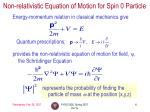Non-relativistic Equation of Motion for Spin 0 Particle

Energy-momentum relation in classical mechanics give

Quantum prescriptions; .

provides the non-relativistic equation of motion for field, y, the Schrödinger Equation

represents the probability of finding the particle of mass m at the position (x,y,z)

PHYS 5326, Spring 2007

Jae Yu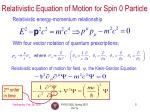2nd order in time

Relativistic Equation of Motion for Spin 0 Particle

Relativistic energy-momentum relationship

With four vector notation of quantum prescriptions;

Relativistic equation of motion for field, y, the Klein-Gordon Equation

PHYS 5326, Spring 2007

Jae Yu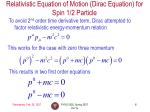To avoid 2nd order time derivative term, Dirac attempted to factor relativistic energy-momentum relation

This works for the case with zero three momentum

This results in two first order equations

PHYS 5326, Spring 2007

Jae Yu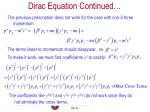Dirac Equation Continued…

The previous prescription does not work for the case with non-0 three momentum

The coefficients like g0=1 and g1= g2= g3=i do not work since they do not eliminate the cross terms.

The terms linear to momentum should disappear, so

To make it work, we must find coefficients gk to satisfy:

PHYS 5326, Spring 2007

Jae Yu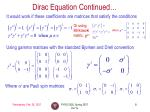Dirac Equation Continued…

It would work if these coefficients are matrices that satisfy the conditions

Or using Minkowski metric, gmn

where

Using gamma matrices with the standard Bjorken and Drell convention

Where si are Pauli spin matrices

PHYS 5326, Spring 2007

Jae Yu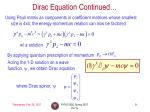Dirac Equation Continued…

Using Pauli matrix as components in coefficient matrices whose smallest size is 4x4, the energy-momentum relation can now be factored

w/ a solution

By applying quantum prescription of momentum

Acting the 1-D solution on a wave function, y, we obtain Dirac equation

where Dirac spinor, y

PHYS 5326, Spring 2007

Jae Yu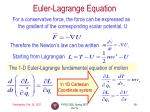Euler-Lagrange Equation

For a conservative force, the force can be expressed as the gradient of the corresponding scalar potential, U

Therefore the Newton’s law can be written .

Starting from Lagrangian

The 1-D Euler-Lagrange fundamental equation of motion

In 1D Cartesian

Coordinate system

PHYS 5326, Spring 2007

Jae YuNote the four vector form

Euler-Lagrange equation in QFT

Unlike particles, field occupies regions of space. Therefore in field theory, the motion is expressed in terms of space and time.

Euler-Larange equation for relativistic fields is, therefore,

PHYS 5326, Spring 2007

Jae YuKlein-Gordon Largangian for scalar (S=0) Field

For a single, scalar field f, the Lagrangian is

Since

and

From the Euler-Largange equation, we obtain

This equation is the Klein-Gordon equation describing a free, scalar particle (spin 0) of mass m.

PHYS 5326, Spring 2007

Jae YuDirac Largangian for Spinor (S=1/2) Field

For a spinor field y, the Lagrangian

Since

and

From the Euler-Largange equation for `y, we obtain

Dirac equation for a particle of spin ½ and mass m.

How’s Euler Lagrangian equation looks like for y?

PHYS 5326, Spring 2007

Jae YuProca Largangian for Vector (S=1) Field

Suppose we take the Lagrangian for a vector field Am

Where Fmn is the field strength tensor in relativistic notation, E and B in Maxwell’s equation form an anti-symmetic second-rank tensor

PHYS 5326, Spring 2007

Jae YuProca Largangian for Vector (S=1) Field

Suppose we take the Lagrangian for a vector field Am

Since

and

From the Euler-Largange equation for Am, we obtain

Proca equation for a particle of spin 1 and mass m.

For m=0, this equation is for an electromagnetic field.

PHYS 5326, Spring 2007

Jae YuLagrangians
• Lagrangians we discussed are concocted to produce desired field equations
• L derived (L=T-V) in classical mechanics
• L taken as axiomatic in field theory
• The Lagrangian for a particular system is not unique
• Can always multiply by a constant
• Or add a divergence
• Since these do not affect field equations due to cancellations

PHYS 5326, Spring 2007

Jae YuHomework
• Prove that Fmn can represent Maxwell’s equations, pg. 225 of Griffith’s book.
• Derive Eq. 11.17 in Griffith’s book
• Due is Wednesday, Mar. 7

PHYS 5326, Spring 2007

Jae Yu